Home News What is baudline? Screenshots Download FAQ Manual Search Solutions Mystery Signal Contact
channel mapping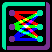The input channel mapping window makes baudline highly configurable.  The mapping of processing operations, transforms, spaces, colors, and routing positions of every channel can be completely controlled.

Click the thumbnail below for a larger image.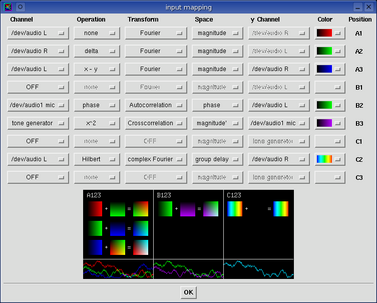Now this is a very complicated looking window, but fortunately most users will never have to use it because baudline automatically configures itself depending on how things are setup in the input devices window.  The general auto configuration defaults are: pack every channel as tightly as possible, no operations, Fourier transform, magnitude display, and a unique, and a constructively summable color ramp.

Note that unlike the input devices window, all the controls here are post buffer, which means they are nondestructive and changing them will retroactively affect all the data in the buffer.  Changes can be made while either recording, paused, or playing.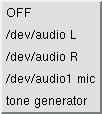Channel
Only enabled channels from the input devices window are visible in this option menu.  The channel selected here will be processed by the following corresponding rules and then displayed at the indicated row position (A1 C2 ...).  Enabled input devices can be chosen multiple times or not at all if desired.  Think of the Channel column as simply a means of mapping any particular input device to any of the 9 possible display positions.Operation
These time-domain operations allow the user to program baudline to perform some simple yet powerful channel and inter-channel analyses.  They work on the input channel sample data and they range from simple arithmetic to more complex DSP operations.  Some operations are dual channel and require a second channel (see the y Channel).

Like all the other channel mapping rules in this window, these operations do not permanently change the buffered data and they are completely reversible.  Note that operations are performed prior to the file saving action.  For example: a stereo file can be converted to a mono file by using the x + y operation.

operation description
none no modification (default)
-x invert, 180° phase shift
| x | absolute value
1 / x reciprocal
sqrt square root, negative values retain sign
x^2 square
x^3 cube
log logarithm, negative values retain sign
clip discard all quantization bits except for sign bit
delta difference coding, X(n) - X(n+1)
| delta | absolute value of the delta operation
-Hz negative frequency, flip frequency axis
Hilbert 90° phase shift
bits convert sample to bits
bit reverse   flip bit order, MSB becomes LSB
flip endian swap bytes, little endian <---> big endian

x + y dual channel, addition
x - y dual channel, subtraction
x * y dual channel, multiplication
x / y dual channel, division
x % y dual channel, modulus
xor dual channel, bitwise exclusive OR
magnitude dual channel, sqrt(x^2 + y^2)
phase dual channel, atan2(x, y)

Note that the majority of these time-domain operations also perform scaling so that sample overflow is avoided.  For example; when the variables x and y are the same the x + y operation will equal x due to scaling.Transform
A transform is a mathematical operation that converts between domains.  The end product of the transform is displayed in baudline's spectrogram and spectrum based windows.  Time domain based samples are the typical transform input but frequency domain samples are an option with specialized hardware.  Other input domains are also possible.  Below are descriptions of the different transforms with accompanying spectrogram snippet images of a sine wave.The Fourier transform (FFT) converts a time domain signal to the frequency domain.  The Fourier transform option is the default and it takes a real time domain signal as input.  The complex Fourier transform performs the Fourier transform on a complex signal which is represented by two inputs: an in-phase (I) component from the Channel menu, and a quadrature (Q) component from the y Channel menu.  For information on automatic complex Fourier mapping see the -quadrature command line option.  The Fourier ratio takes the difference between two Fourier transforms of two different input channels.The blip Fourier transform utilizes a blind phase locking algorithm built on a new analysis primitive called the blip(let) to enhance the spectral display in both magnitude and phase spaces.  This does two valuable things.  First, the spectral resolution is improved in the magnitude space which is ideal for observing amplitude beating while deep zooming down to the sample level. Second, spinning phase is now locked which allows the phase space to contain visibly useful information.  A focus parameter in the process menu allows for fine tuning the algorithm on a signal by space by zoom basis.  For more information see the blip Fourier preview and blip BPSK demodulation blog posts.The transfer function is a dual channel transform that is useful for observing the relationship between the input and output of a system.  It is a linear mapping of the Laplace transform of the input X(f) to the output Y(f) in the formula Y(f) = H(f) * X(f) where H(f) is the transfer function and the "*" operator is convolution.  Another way of looking at H(f) is that it is a frequency domain filter that shows how two channels are related.  Magnitude and phase space both contain useful information.The impulse response is a dual channel transform that is a time domain representation of the transfer function described above.  The input X(t) is related to the output Y(t) in the formula Y(t) = H(t) * X(t) where H(t) is the impulse response and the "*" operator is convolution.  The impulse response can be thought of as the taps of an FIR filter.The Fourier ratio transform compares the magnitude frequency response between two channels.  Function-wise it basically is the transfer function in magnitude space.  It currently has better averaging qualities than the transfer function transform but that will be remedied in a future baudline release.The Correlation transforms are a measure of similarity.  The cross-correlation transform shows the similarity between two different input channels with the output results interpreted as a time lag measurement.  The Autocorrelation transform shows the self similarity of a signal which is useful because it can remove random noise.  The delta operation is often used with both correlation transforms as a quick and simple technique for reducing a strong DC offset or low frequency 50/60 Hz hum.  The Autocorrelation transform can also be utilized as a form of waveform trigger lock mechanism.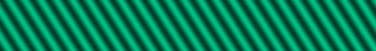The sample Raster transform is a method of observing a signal's raw sample structure.  The 16-bit samples are displayed side-by-side on horizontal raster rows.  The sample Raster works a lot like the horizontal scan line of a television, in fact NTSC and PAL images can be displayed with a high enough sample rate (bandwidth) and line width setting.  The overlap slide size setting of the Scroll Control window is critical for proper raster slice alignment.  The stacked raster slices are also a way of investigating periodic behavior.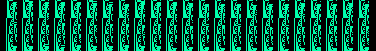The bit Raster transform is a method of looking at the bit structure of the raw sample data.  Individual binary bits are displayed in MSB first order on horizontal raster rows.  Like the sample Raster transform, the raster width can be adjusted with the overlap side size of the Scroll Control window.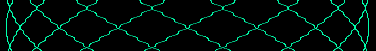The Histogram transform is a method of looking at the probability distribution of the sample amplitudes.  It is a vertical slice representation of the Histogram window and it is a useful viewpoint for investigating both time domain and raw binary data files.

The OFF transform simply disables the spectral display for that channel which is a way of doing a Waveform or Histogram window only mapping.  It is also a useful way for reducing CPU load.

All of the spectrogram snippets above were generated with a basic sine wave and the respective transform.  Either magnitude or linear space was used for all transforms expect for the blip Fourier and transfer function which used phase space.  The dual channel transforms used the clip operation to create two unique channels.

Note that the complex Fourier, Fourier ratio, transfer function, impulse response, and cross-correlation transforms are dual channel and they all use the y Channel method for selecting the second input channel.  Either the Operation menu or the Transform menu can use the y Channel, not both at the same time.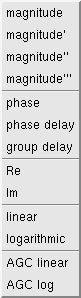Space
While the Transform changes domains, the Space option translates the domain data into geometries.  This geometrical transposition of space allows graphical visualization and it creates units such as dB, radians, bits, and samples.  The Fourier transforms utilize the magnitude, phase, and complex component spaces while the Correlation, Raster, and Histogram transforms utilize the linear, logarithmic, and AGC combination spaces.

The magnitude space, also known as the logarithm of the modulus or distance, is dB in the spectrum window.  The magnitude option is the default space.  The magnitude''' primes are crude 1st, 2nd, and 3rd derivatives of the magnitude of each frequency bin in respect to time.

The phase space is an angular measure of the complex frequency components.  The phase option has units of radians and it is a phase angle representation of a given frequency relative to the start of the spectral window.  The phase delay option is the phase response as a function of frequency.  In more technical terms it is the time delay experienced by a sinusoid of a particular frequency as it passes through the system.  group delay is a derivative of the phase response which is how the phase changes as a function of frequency.  The phase spaces need to be locked to a trigger, otherwise they will be "free spinning" and locked only to the sliding spectral window which is quite arbitrary.

The Re and Im space options are logarithmic representations of the frequency domain's complex real and imaginary components.  The scaling is logarithmic and negative values retain their sign.  The Re and Im spaces are valuable educational tools for examining the raw complex frequency data and in understanding how the magnitude and phase spaces work.

The linear and logarithmic spaces are amplitude mappings used by the Correlation, Raster, and Histogram transforms.  The linear space option is a flat mapping.  The logarithmic space option is log mapping, like dB, with the added caveat that negative values retain their sign.  The AGC linear and AGC logarithmic options utilize an AGC (Automatic Gain Control) to dynamically adjust the amplitude scaling such that weak signals are visible.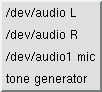y Channel
This menu selects the second input channel used either in the Operation or Transform options that use dual channels.  Because of this sharing, you cannot have a combination such as the cross-correlation of signal A and signal A + B.Color
The default colors (red, green, blue, purple, and seafoam) are automatically chosen based on the number of enabled channels so that there are no color mixing problems.  A single input channel in a positional column defaults to seafoam (also know as baudline green).  Two input channels default to purple and green, whereas three input channels default to red, green, and blue since this is the only mixture that will result in clean uncorrupted color mixing.

See the black color mixing box below.  Many other workable combinations are possible.  This palette lets the user manually change color ramps.  Note that the three color ramps at the bottom are the user custom configurable color ramps which can be modified with the color picker window.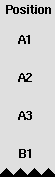Position
The input Channel of any given row is mapped to be displayed at this screen position.  There are three display columns: A, B, C, and there are three overlapping mixed channels per column labeled as 1, 2, 3.  Together this pair forms a coordinate which is the display position.  The positions are fixed but the input channels can be moved around freely.  See the black color mixing box below for an illustration of the physical layout of position mapping for A{123}, B{123}, and C{123}.black color mixing boxThe above figure shows the physical layout of the positions and it shows how the chosen color ramps add together.  Choosing color ramps that do not blend correctly will result in blockiness in the sum of the color equations (see the example to the right).  This would be bad, and checking the color mixing blocks for damage will prevent mixing problems in the spectrogram window at different amplitude (dB) levels. The black color mixing box can also be used as a visual status showing which channels are enabled, and their location in the spectral display.